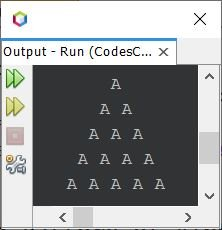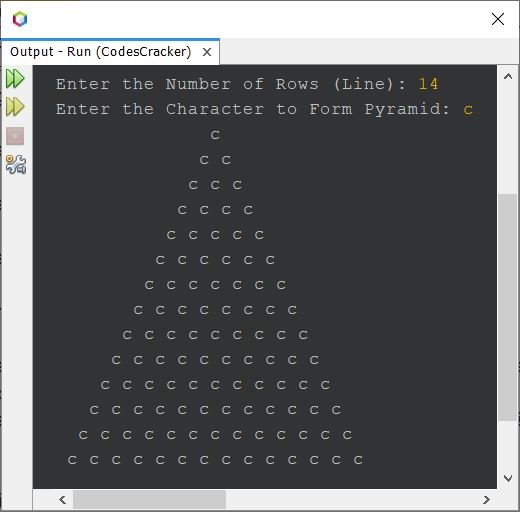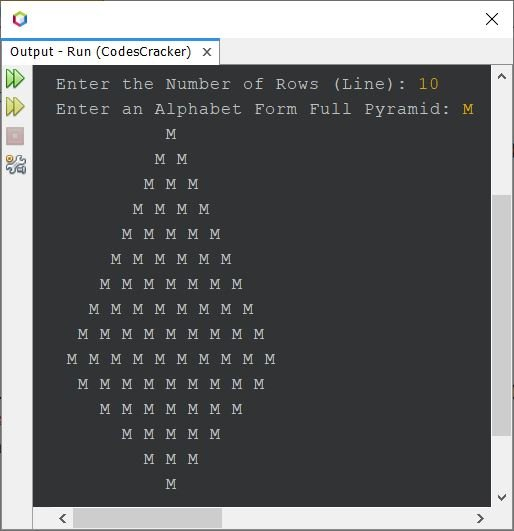# Java Program to Print Pyramid Pattern of Alphabet

This article covers pyramid pattern of alphabet in Java. The three most famous pyramid pattern of alphabet in Java, are covered in this article.

## Pyramid Pattern of Alphabets in Java

The question is, write a Java program to print pyramid pattern of alphabet character. The program given below is its answer:

```public class CodesCracker
{
public static void main(String[] args)
{
int i, space, j;
char alphabet = 'A';
for(i=0; i<5; i++)
{
for(space=i; space<5; space++)
System.out.print(" ");
for(j=0; j<(i+1); j++)
System.out.print(alphabet+ " ");
System.out.print("\n");
}
}
}```

The snapshot given below shows the sample output produced by above program on printing of a pyramid pattern using alphabet character:Now the program given below is the modified version of the previous program. This program allows user to define the size of pyramid, along with the character to form the pyramid, with.

```import java.util.Scanner;

public class CodesCracker
{
public static void main(String[] args)
{
Scanner s = new Scanner(System.in);

System.out.print("Enter the Number of Rows (Line): ");
int row = s.nextInt();
System.out.print("Enter the Character to Form Pyramid: ");
char ch = s.next().charAt(0);

for(int i=0; i<row; i++)
{
for(int space=i; space<row; space++)
System.out.print(" ");
for(int j=0; j<(i+1); j++)
System.out.print(ch+ " ");
System.out.print("\n");
}
}
}```

The sample run of above program with user input c is shown in the snapshot given below:## Print Full Pyramid of Alphabet in Java

The pyramid of alphabet, created using this program, looks like the actual pyramid. Therefore, I've called this as the full pyramid of alphabet.

```import java.util.Scanner;

public class CodesCracker
{
public static void main(String[] args)
{
int i, row, s, j;
char alphabet;
Scanner s = new Scanner(System.in);

System.out.print("Enter the Number of Rows (Line): ");
row = s.nextInt();
System.out.print("Enter an Alphabet Form Full Pyramid: ");
alphabet = s.next().charAt(0);

for(i=0; i<row; i++)
{
for(s=i; s<row; s++)
System.out.print(" ");
for(j=0; j<(i+1); j++)
System.out.print(alphabet+ " ");
System.out.print("\n");
}
for(i=row; i>0; i=(i-2))
{
for(s=row; s>=(i-1); s--)
System.out.print(" ");
for(j=(i-1); j>0; j--)
System.out.print(alphabet+ " ");
System.out.print("\n");
}
}
}```

Here is its sample run with user input 10 as row size and M as alphabet to form a full pyramid using this alphabet:Note - For more pyramid pattern using alphabet, refer to Pyramid Pattern of Stars in Java. The only change to do, is replace the star with desired alphabet character.

Java Online Test

« Previous Program Next Program »